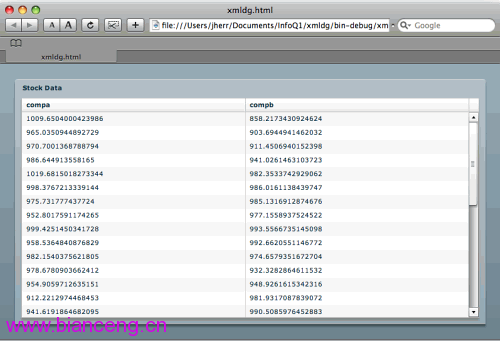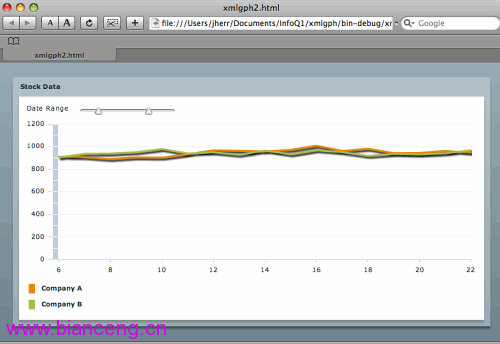﻿Flex与JSON及XML的互操作

# Flex与JSON及XML的互操作

Flex之于Java，就像美丽之于大脑，或者还有别的说法？谁能告诉我？我所知道的是，Flex和Java真的是能配合得很好，能创建出难以置信 的富 Internet应用（RIA）。你会问Flex是什么？Flex是一个开源框架，你可以通过基于标签的MXML语言（以及ActionScript 3）来构建Flash 应用。

http://www.jackherrington.com/screencasts/FlexAndJSON.mov

XML示例由列表1中显示的简单JSP文件开始：

`列表1. xml.jsp double compa = 1000.0; double compb = 900.0; for (int i = 0; i<=30; i++) { compa += ( Math.random() * 100 ) - 50; compb += ( Math.random() * 100 ) - 50; ]]> i compa compb ]]> `

`% curl "http://localhost:8080/jsp-examples/flexds/xml.jsp" 0966.429108587301 920.7133933216961 ...``列表2. xmldg.mxml </mx:application　xmlns:mx="http:>`

xmldg应用程序代 码有两个主要的组件。第一个是标签，它告诉Flex这是个XML数据源，并提供了URL。这样就会创建一个叫做stockData（由id属 性指定）的局部变量，而组件可以把它当作dataProvider来使用。`列表3. xmldg2.mxml <mx:panel title="Stock Data" width="100%" height="100%" layout="vertical" paddingBottom="10" paddingLeft="10" paddingRight="10" paddingTop="10"> `

{stockData..day.(num >= daySlider.value )}`列表4. xmlgph.mxml <mx:panel title="Stock Data" width="100%" height="100%" layout="vertical" paddingBottom="10" paddingLeft="10" paddingRight="10" paddingTop="10"> <mx:linechart id="chart" dataprovider="{stockData..day.(num >= daySlider.value )}" width="100%" height="100%"> `` <mx:panel title="Stock Data " width="100% " height="100% " layout="vertical " paddingBottom="10 " paddingLeft="10 " paddingRight="10 " paddingTop="10 "> <mx:hslider minimum="0 " maximum="30 " id="daySlider " snapinterval="1 " thumbCount="2 " values="[0,30] " /> <mx:linechart id="chart" dataProvider="{stockData..day.(num>=daySlider.values && num<=daySlider.values)}" width="100%" height="100%"> ``列表6. json.jsp [ double compa = 1000.0; double compb = 900.0; for (int i = 0; i<=30; i++) { compa += ( Math.random() * 100 ) - 50; compb += ( Math.random() * 100 ) - 50; if ( i > 0 ) out.print( "," ); ]]> {"compa":compa,"compb":<jsp:expres  sion>compb} ]]> ] `

`% curl "http://localhost:8080/jsp-examples/flexds/json.jsp" [{"compa":992.2139849199265,"compb":939.89135379532}, ...]`

`列表7. jsondg.mxml <mx:application xmlns:mx="http://www.adobe.com/2006/mxml" layout="vertical" creationComplete="jsonservice.send ()"> import mx.rpc.events.ResultEvent; import com.adobe.serialization.json.JSONDecoder; private function onJSONResult( event:ResultEvent ) : void { var data:String = event.result.toString(); data = data.replace( /\s/g, '' ); var jd:JSONDecoder = new JSONDecoder( data ); dg.dataProvider = jd.getValue(); } ]]> <mx:httpservice id="jsonservice" url="http://localhost:8080/jsp- examples/flexds/json.jsp" resultFormat="text" result="onJSONResult(event)" /> `